# CEOE Middle Level/Intermediate Math: Circles & Arc of a Circle Chapter Exam

Exam Instructions:

Choose your answers to the questions and click 'Next' to see the next set of questions. You can skip questions if you would like and come back to them later with the yellow "Go To First Skipped Question" button. When you have completed the practice exam, a green submit button will appear. Click it to see your results. Good luck!

### Page 1

#### Question 4 4. If arc AC is 122 degrees and arc BC is 192 degrees, what is the measure of angle ACB?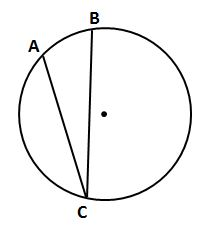### Page 2

#### Question 8 8. If arc BC is 84 degrees, what is the measure of angle ACB?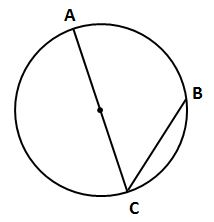### Page 3

#### Question 12 12. In the picture below, if AE is 10, AB is 14 and ED is 16, what is the length of CE?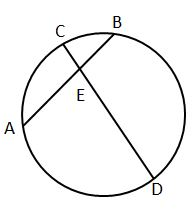#### Question 14 14. In the picture below, if major arc BC is 258 degrees and minor arc BC is 102 degrees, what is the measure of angle BAC?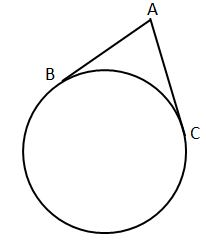#### Question 15 15. If arc AC is 106 degrees and arc BC is 82 degrees, what is the measure of angle ACB?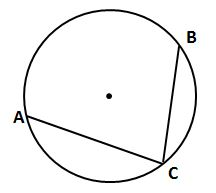### Page 4

#### Question 17 17. If the measure of central angle AOB is 62 degrees, what is the measure of inscribed angle ACB?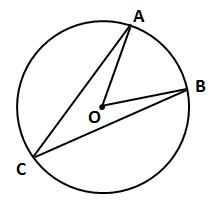#### Question 20 20. If angle ACB is 34 degrees, what is the measure of angle ADB?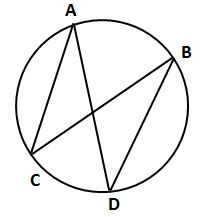### Page 5

#### Question 25 25. In the picture below, if AE is 8, CE is 12 and ED is 5, what is the length of EB?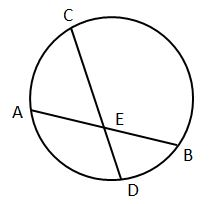### Page 6

#### Question 27 27. In the picture below, if AC is 14 and AB is 10, what is the length of AD?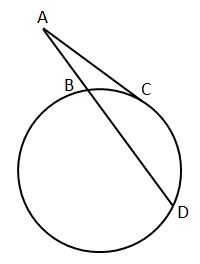#### Question 28 28. In the picture below, if arc AC is 146 degrees and arc BD is 32 degrees, what is the measure of angle AEC?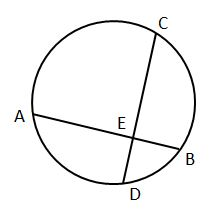#### Question 29 29. If arc AB is 72 degrees, what is the measure of angle ACB?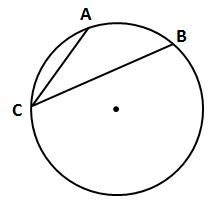#### CEOE Middle Level/Intermediate Math: Circles & Arc of a Circle Chapter Exam Instructions

Choose your answers to the questions and click 'Next' to see the next set of questions. You can skip questions if you would like and come back to them later with the yellow "Go To First Skipped Question" button. When you have completed the practice exam, a green submit button will appear. Click it to see your results. Good luck!

Support